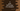# C program to print the ASCII value of a character### C program to print the ASCII value of a character :

American Standard Code for Information Interexchange or ASCII is a character encoding standard or a value between 0 and 127, that determines a character variable. For example, ASCII value of ‘A’ is 65. In this example program, we will learn how to print the ASCII value of a character in ‘C’ programming language.

### C Program code :

``````#include<stdio.h>

int main(){
//1
char ch;

//2
printf("Enter a character : ");
scanf("%c",&ch);

//3
printf("ASCII value of %c is %d \n",ch,ch);

}``````

### Explanation :

The commented numbers above indicate the steps number below :

1. First, create one character variable ‘ch’ to store the character value.
2. Ask the user to enter a character. And save the character in ‘ch’.
3. Now print the character and ASCII value of the character. We are using %d to print the ASCII value and _%c to print the character. _

### Output :

``````Enter a character : B
ASCII value of B is 66

Enter a character : A
ASCII value of A is 65

Enter a character : a
ASCII value of a is 97

Enter a character : b
ASCII value of b is 98

Enter a character : Z
ASCII value of Z is 90``````

You might also like :